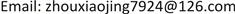﻿ “工匠型”创新人才培养模式下学科师资队伍评价体系研究 Research on Teacher Faculty Evaluation System Based on Innovative Talent Training Mode of Artisan Type

Operations Research and Fuzziology
Vol. 09  No. 01 ( 2019 ), Article ID: 28701 , 7 pages
10.12677/ORF.2019.91006

Research on Teacher Faculty Evaluation System Based on Innovative Talent Training Mode of Artisan Type

Jinyan Zhang1, Yuan Yuan2, Mingcong Zhang3, Xiaojing Zhou1, Yanfeng Li1, Xiaoqiu Yu1

1College of Science, Heilongjiang Bayi Agricultural University, Daqing Heilongjiang

2College of Life Science Technology, Heilongjiang Bayi Agricultural University, Daqing Heilongjiang

3College of Agriculture, Heilongjiang Bayi Agricultural University, Daqing HeilongjiangReceived: Jan. 9th, 2019; accepted: Jan. 22nd, 2019; published: Jan. 29th, 2019ABSTRACT

Combining with the established “artisan-type” innovative talent training model, this paper constructed a practical evaluation index system for teaching staff, then the factor analysis method was applied for actual measurement analysis. Finally the rectification strategy was proposed based on the results.

Keywords:Teaching Staff, Evaluation, Factor Analysis

“工匠型”创新人才培养模式下学科师资队伍评价体系研究

1黑龙江八一农垦大学理学院，黑龙江 大庆

2黑龙江八一农垦大学生命学院，黑龙江 大庆

3黑龙江八一农垦大学农学院，黑龙江 大庆1. 引言

2. 师资队伍评价指标体系构建

2.1. 评价指标体系构建依据

2.2. 指标体系框架

Table 2. Second and third index of researchingTable 3. Second and third index of management and service

3. 师资队伍评价指标体系分析Table 4. Total variance of principal component interpreted

Table 5. Coefficients of Principal component of 14 secondary indicators of 3 majors

$\begin{array}{c}{y}_{1}=0.976{x}_{1}^{*}-0.981{x}_{2}^{*}-0.890{x}_{3}^{*}-0.994{x}_{4}^{*}-0.971{x}_{5}^{*}\\ +0.885{x}_{6}^{*}+0.885{x}_{7}^{*}+0.762{x}_{8}^{*}+0.266{x}_{9}^{*}+0.636{x}_{10}^{*}\\ +0.774{x}_{11}^{*}+0.593{x}_{12}^{*}+0.636{x}_{13}^{*}+0.954{x}_{14}^{*}\end{array}$

$\begin{array}{c}{y}_{2}=0.129{x}_{1}^{*}-0.188{x}_{2}^{*}+0.369{x}_{3}^{*}+0.069{x}_{4}^{*}-0.145{x}_{5}^{*}\\ -0.418{x}_{6}^{*}-0.418.{x}_{7}^{*}+0.584{x}_{8}^{*}+0.738{x}_{9}^{*}+0.183{x}_{10}^{*}\\ -0.487{x}_{11}^{*}+0.690{x}_{12}^{*}+0.183{x}_{13}^{*}-0.232{x}_{14}^{*}\end{array}$

$\begin{array}{c}{y}_{3}=-0.173{x}_{1}^{*}-0.038{x}_{2}^{*}+0.269{x}_{3}^{*}-0.086{x}_{4}^{*}-0.189{x}_{5}^{*}\\ +0.204{x}_{6}^{*}+0.204{x}_{7}^{*}-0.279{x}_{8}^{*}+0.620{x}_{9}^{*}-0.750{x}_{10}^{*}\\ +0.404{x}_{11}^{*}+0.415{x}_{12}^{*}-0.750{x}_{13}^{*}+0.192{x}_{14}^{*}\end{array}$Table 6. Score of Teacher team evaluation index of major 1Table 7. Score of Teacher team evaluation index of major 2Table 8. Score of Teacher team evaluation index of major 3

4. 结论

Research on Teacher Faculty Evaluation System Based on Innovative Talent Training Mode of Artisan Type[J]. 运筹与模糊学, 2019, 09(01): 45-51. https://doi.org/10.12677/ORF.2019.91006

1. 1. 史万兵, 杨慧. 高等学校教师科研绩效评价方法与研究[J]. 高教探索, 2014(6): 112-117.

2. 2. 刘莉莉. 高校师资队伍结构优化及其对策研究[J]. 东南大学学报(哲学社会科学版), 2010, 12(6): 126-129.

3. 3. 房国忠, 孙杏梅, 杨雪. 高校教师综合绩效评价系统设计[J]. 东北师大学报(哲学社会科学版), 2006(3): 156-160.

4. 4. 陈鹏, 陈志鸿, 张祖新, 等. 美国高校师资管理目标及外化评价指标研究[J]. 中国高教研究, 2009(1): 36-40.

5. 5. 李扬裕, 何东进. 高校师资队伍学缘结构评价和预测方法研究[J]. 福建农林大学学报(哲学社会科学版), 2010, 13(5): 102-105.

6. 6. 李科. 教师全面评价系统在美国大学的应用与实践[J]. 职业技术教育, 2014, 35(4): 89-93.

7. 7. 张宁. 高校教师绩效评价的定性分析及数学模型[J]. 教育思想研究, 2012: 81-84.

8. 8. 熊凤山, 卢凤刚, 邓明净, 等. 基于动态化和差异化的高校教师绩效评价体系研究[J]. 河北农业大学学报(农林教育版), 2011, 13(1): 1-3, 7.

9. 9. 陈绍辉, 金喜在. 高校教师绩效评价的内涵、维度与方法[J]. 社会科学家, 2018, 2(2): 118-120.

10. 10. 丁政, 吴福根, 徐小明. 高校实验教师评价体系的构建与实施[J]. 实验技术与管理, 2017, 31(4): 228-234.